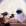MathematicsOpenStudy (anonymous):

two sides of a quadrilateral have the same length, x, while the other two sides have the same length, both being the next consecutive off integer. Write the sum of these lengths. Then simplify the expression. more, if the perimeter of the figure is 8 units, then please find the length of each side of the provided quadrilateralOpenStudy (anonymous):

So two sides are x, and the other two are x+1, this equates to x + x + x+1 + x+1 which equals 4x + 2 If you set this equal to 8, 4x + 2 = 8, 4x = 6, x = 1.5, then that means two sides are 1.5 units and the other are 2.5 unitsOpenStudy (anonymous):

thank you so muchkittybasil: \[\frac{3}{14}+\frac{10}{21}\div \left(\frac{3}{7}\right)\left(\frac{9}{4}\right)stephaniepargas12: reveal the following passages. how does the poet's use of alliteration influenceEsmeperez2003: determine the length of the object shownkainpate: Jerry deposits $5,000 at the end of each year in an account earning 2.25% interest, compounded annually. 47 minutes ago 0 Replies 0 Medalsidontcare369: Marjorie is trying to determine the identity of an unknown substance. After running tests, she determines that the substance is insoluble in CCl4 with a den 1 hour ago 1 Reply 0 Medalscburry: Which statement best describes the difference between the way the poets address t 1 hour ago 0 Replies 0 Medalskris062105: Find MN such that LN = 5.7. 1 hour ago 0 Replies 0 MedalsBlueStar: Hi, please show me how to solve this problem. A compound consists of carbon, hydrogen, oxygen and bromine. 1 hour ago 0 Replies 0 MedalsOlga11024: Name the kind or kinds of symmetry the following figure has: point, line, plane, or none. 2 hours ago 0 Replies 0 Medalsferdean: Blake Turner, a pharmacy technician, earns an annual gross pay of$41,300. He is married with two dependents, and his exemptions total \$8,000.